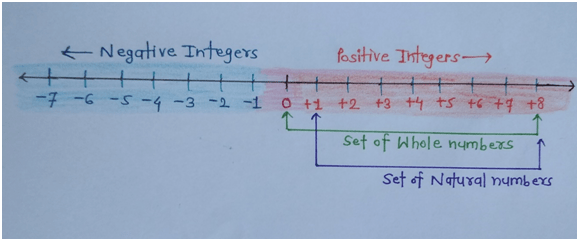# Prime numbers

• In mathematics numbers are classified according to the properties shown by particular group of numbers. There is a interesting thing behind each set of numbers we have note it only. In short we say that math is started from numbers only.
• The set of integers includes all positive and negative integers including zero also. It is the largest set of numbers which includes natural numbers, whole numbers, prime numbers, composite numbers, even numbers and odd numbers also.• The set of natural number N = {1, 2, 3, 4, 5…} is classified into prime numbers and composite numbers.

Note: One is neither prime nor composite number.## Prime numbers:

• The prime numbers are the natural numbers which are having factors one and the number itself i.e. the prime numbers are divisible by one and the number itself.
• If we divide them by other number then the remainder will not be zero.
• The prime numbers starts from 2, 3, 5, 7, 11,…. And so on.
• The prime numbers are not expressed in the product form of other numbers except one and itself.

#### For example:

• 2 is the prime number which is expressed as 2= 2*1 only
• It has no other factors, hence it is the first prime number.

• 3 is the prime number which is expressed as 3 = 3*1 only.
• It has no other factors, hence it is the second prime number.
• If we divide 3 by 2 then we will not get the quotient in the form of whole number and also remainder will not be zero.
• Hence 3 is the prime number which is odd number also.

• 5 is the prime number which is expressed as 5 = 5*1 only.
• It has no other factors than 1 and itself, hence it is the third prime number.
• If we divide 5 by any other number other than 1 and 5 then we will not get quotient as whole number it will be in fraction only and also remainder will not be zero.
• Such as if we divide 5 by 2 then the quotient will be 2.5 and remainder is zero.
• Hence here quotient is in fraction, so 5 is divisible by 1 and itself completely.

### Prime numbers between 1 and  20:

The prime numbers between 1 and 20 are 2, 3, 5, 7, 11, 13, 17, and 19. All these are the prime numbers as they are having the factors one and itself. All these prime numbers are natural numbers also.

For example:

• The number 11 is only completely divisible by 1 and itself, hence 11 is the prime number. And 11 is the odd prime number.
• The number 13 also only divisible by 1 and 13 completely, hence it is also the prime number. And 13 is the odd prime number.
• Similarly, 17 and 19 are also divisible by only 1 and itself, so they are the prime numbers and odd prime numbers mainly.

### Lowest prime number:

• As the prime numbers starts from 2, 3, 5, 7, 11,… and so on. The lowest prime number is the 2 which is the natural number.
• Because 2 cannot be expressed in the form of product of numbers other than 2 and 1.
• Since, 2 = 2* 1
• Hence, it is the first and lowest prime number.

#### 1 and 0 are prime or not, why?

• 1 and 0 are neither prime number nor composite number because the number 1 has only one factor which is itself i.e. 1. Hence, 1 cannot be considered as the prime number.
• And zero is not the prime number also.

### Prime numbers between 1 and 100:

• The prime numbers between 1 and 100 are 2, 3, 5, 7, 11, 13, 17, 19, 23, 29, 31, 41, 43, 47, 53, 59, 61, 67, 71, 73, 79, 83, 89, and 97.
• All these are the prime numbers as they are having factors only 1 and the number itself.
• If we observe that except the prime number 2 all the other prime numbers are odd numbers only.

### Even and odd prime numbers:

• The first and lowest prime number is 2, which is the only one even prime number.
• All the other prime number except 2 all are the odd prime numbers only.
• 3, 5, 7, 11, 13, 17, 19, 23, 29, 31, 41, 43, 47,…. All are the odd prime numbers only.

### Key points to note:

1) The interesting thing is that every prime number greater than 3 can be expressed in the form (6n+1) or (6n – 1), but the converse is not true.

For example:

• The first prime number greater than 3 is 5, which is expressed as

5 = 6n – 1 = 6*1 – 1 = 6-1= 5

• 7 = 6n + 1= 6*1 + 1= 6 + 1 =7
• 11 = 6*2 – 1 = 12 – 1 = 11
• 13 = 6*2 + 1 = 12 + 1 = 13
• In this way we can express the prime numbers greater than 3 in the form of (6n + 1) or (6n – 1).

Note: The numbers in the form written (6n + 1) or (6n – 1) are not always the prime numbers.

Number Names MCQ

Number System MCQ

Ordinal Numbers MCQ

Consecutive Numbers MCQ

Integers MCQ

Natural Numbers MCQ

Whole Numbers MCQ

Even Numbers MCQ

Updated: November 3, 2022 — 2:50 pm